# Circle Theorems

Some interesting things about angles and circles.

## Inscribed Angle

First off, a definition:

Inscribed Angle: an angle made from points sitting on the circle's circumference.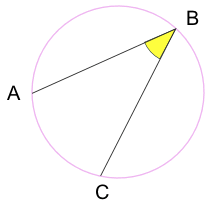A and C are "end points"
B is the "apex point"

Play with it here:

When you move point "B", what happens to the angle?

## Inscribed Angle Theorems

An inscribed angle is half of the central angle 2a°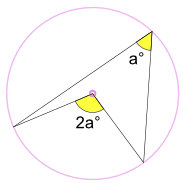(Called the Angle at the Center Theorem)

And (keeping the end points fixed) ...

... the angle is always the same,
no matter where it is on the same arc between end points: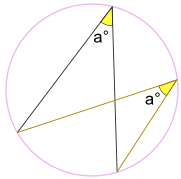Angle a° is the same.
(Called the Angles Subtended by Same Arc Theorem)

### Example: What is the size of Angle POQ? (O is circle's center)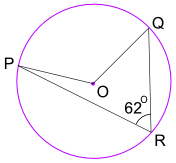Angle POQ = 2 × Angle PRQ = 2 × 62° = 124°

### Example: What is the size of Angle CBX?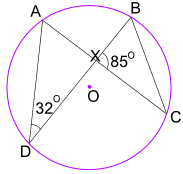Angle ADB = 32° also equals Angle ACB.

And Angle ACB also equals Angle XCB.

So in triangle BXC we know Angle BXC = 85°, and Angle XCB = 32°

Now use angles of a triangle add to 180° :

Angle CBX + Angle BXC + Angle XCB = 180°
Angle CBX + 85° + 32° = 180°
Angle CBX = 63°

## Angle in a Semicircle (Thales' Theorem)

An angle inscribed across a circle's diameter is always a right angle:(The end points are either end of a circle's diameter,
the apex point can be anywhere on the circumference.)

 Why? Because: The inscribed angle 90° is half of the central angle 180° (Using "Angle at the Center Theorem" above)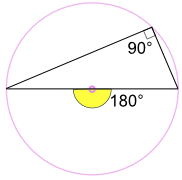### Another Good Reason Why It Works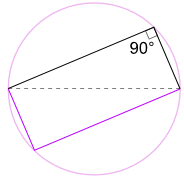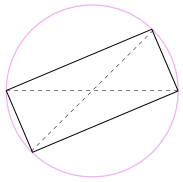We could also rotate the shape around 180° to make a rectangle!

It is a rectangle, because all sides are parallel, and both diagonals are equal.

And so its internal angles are all right angles (90°).So there we go! No matter where that angle is
on the circumference, it is always 90°

### Example: What is the size of Angle BAC?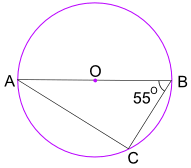The Angle in the Semicircle Theorem tells us that Angle ACB = 90°

Now use angles of a triangle add to 180° to find Angle BAC:

Angle BAC + 55° + 90° = 180°
Angle BAC = 35°

### Finding a Circle's CenterWe can use this idea to find a circle's center:

• draw a right angle from anywhere on the circle's circumference, then draw the diameter where the two legs hit the circle
• do that again but for a different diameter

Where the diameters cross is the center!

### Drawing a Circle

When we know two opposite points on a circle we can draw that circle.

Put some pins or nails on those points and use a builder's square like this:A "Cyclic" Quadrilateral has every vertex on a circle's circumference:A Cyclic Quadrilateral's opposite angles add to 180°: a + c = 180° b + d = 180°### Example: What is the size of Angle WXY?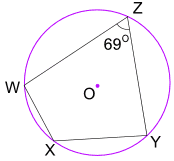Angle WZY + Angle WXY = 180°
69° + Angle WXY = 180°
Angle WXY = 111°## Tangent Angle

A tangent line just touches a circle at one point.

It always forms a right angle with the circle's radius.